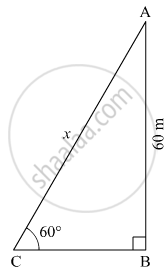# A Kite is Flying at a Height of 60 M Above the Ground. the String Attached to the Kite is Tied at the Ground. It Makes an Angle of 60° with the Ground. Assuming that the String is Straight, - Geometry

#### Question

A kite is flying at a height of 60 m above the ground. The string attached to the kite is tied at the ground. It makes an angle of 60° with the ground. Assuming that the string is straight, find the length of the string.

$\left( \sqrt{3} = 1 . 73 \right)$

#### Solution

Let AB be the height of kite above the ground and C be the position of the string attached to the kite which is tied at the ground.

Suppose the length of the string be x m.Here, AB = 60 m and ∠ACB = 60º
In right ∆ABC,
$\sin60^\circ = \frac{AB}{AC}$
$\Rightarrow \frac{\sqrt{3}}{2} = \frac{60}{x}$
$\Rightarrow x = \frac{120}{\sqrt{3}} = 40\sqrt{3}$
$\Rightarrow x = 40 \times 1 . 73 = 69 . 2 m$

Thus, the length of the string is 69.2 m.

Is there an error in this question or solution?

#### APPEARS IN

Balbharati Mathematics 2 Geometry 10th Standard SSC Maharashtra State Board
Chapter 6 Trigonometry
Practice Set 6.2 | Q 6 | Page 137

#### Video TutorialsVIEW ALL 

A Kite is Flying at a Height of 60 M Above the Ground. the String Attached to the Kite is Tied at the Ground. It Makes an Angle of 60° with the Ground. Assuming that the String is Straight, Concept: Heights and Distances.